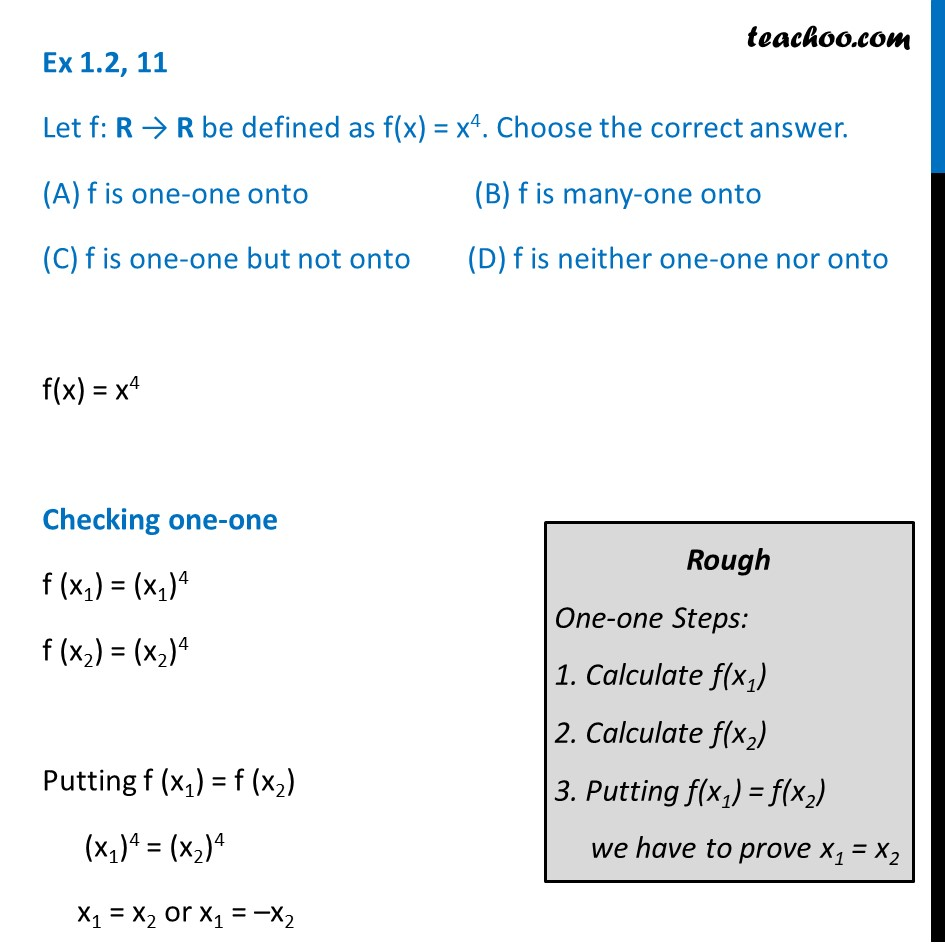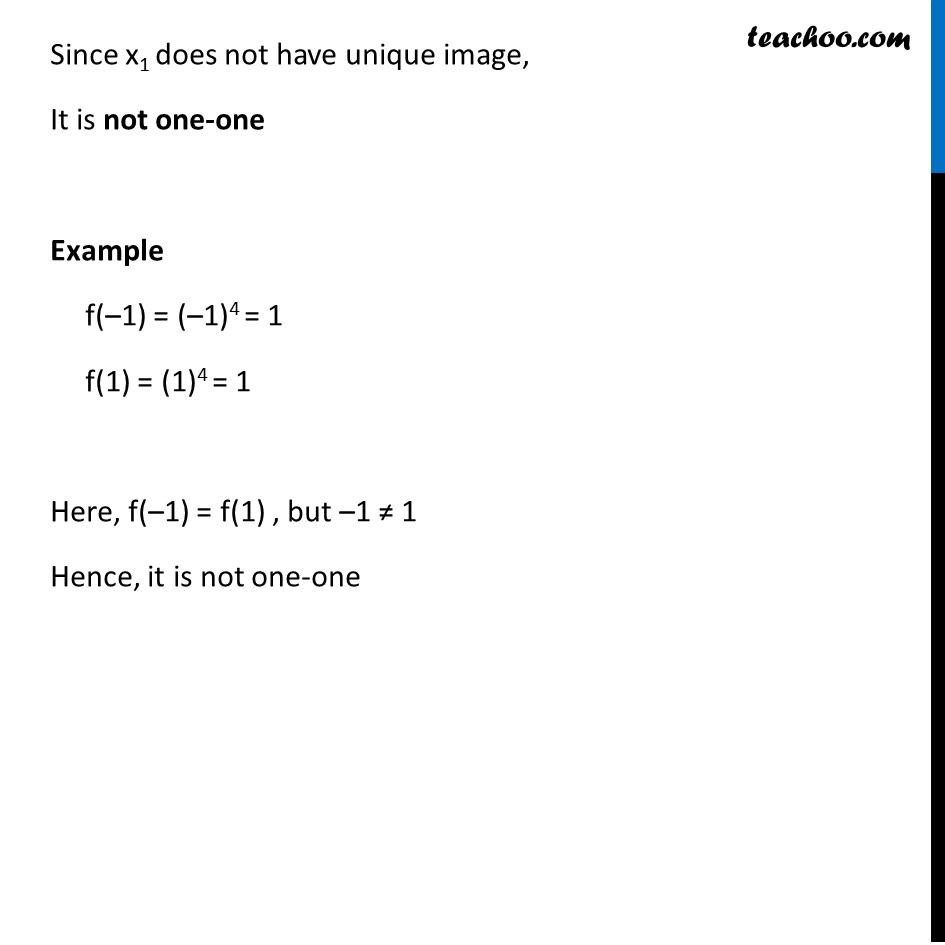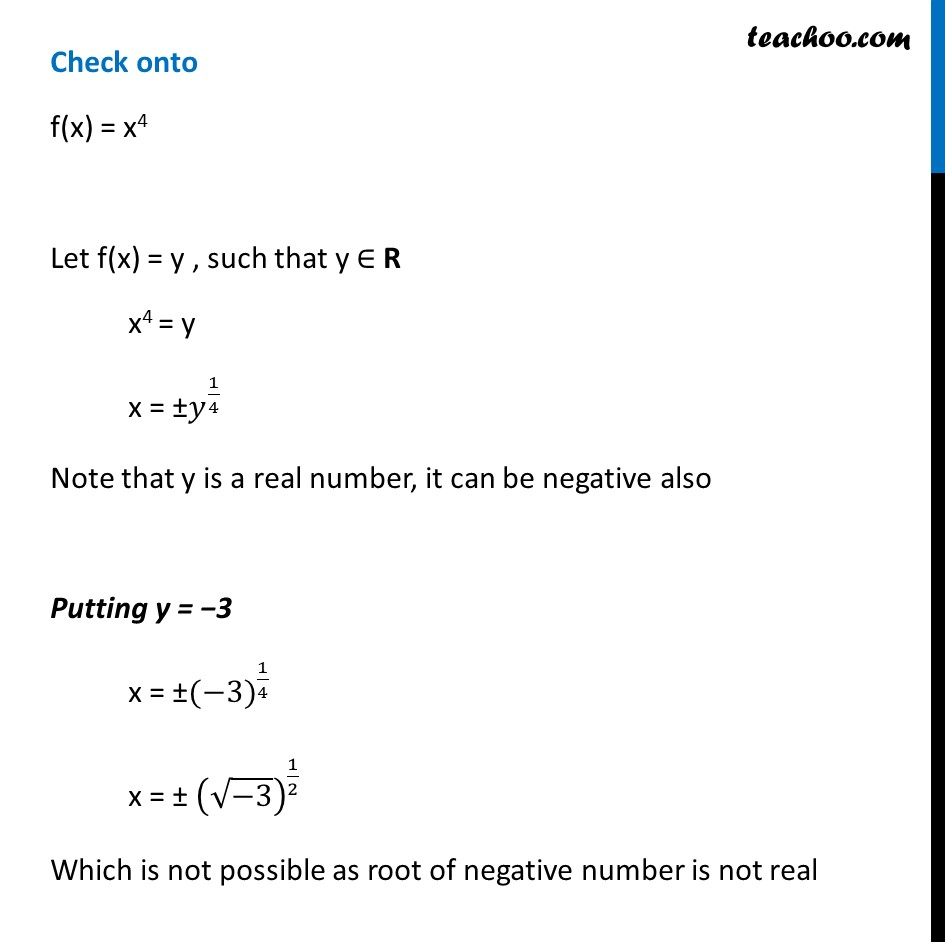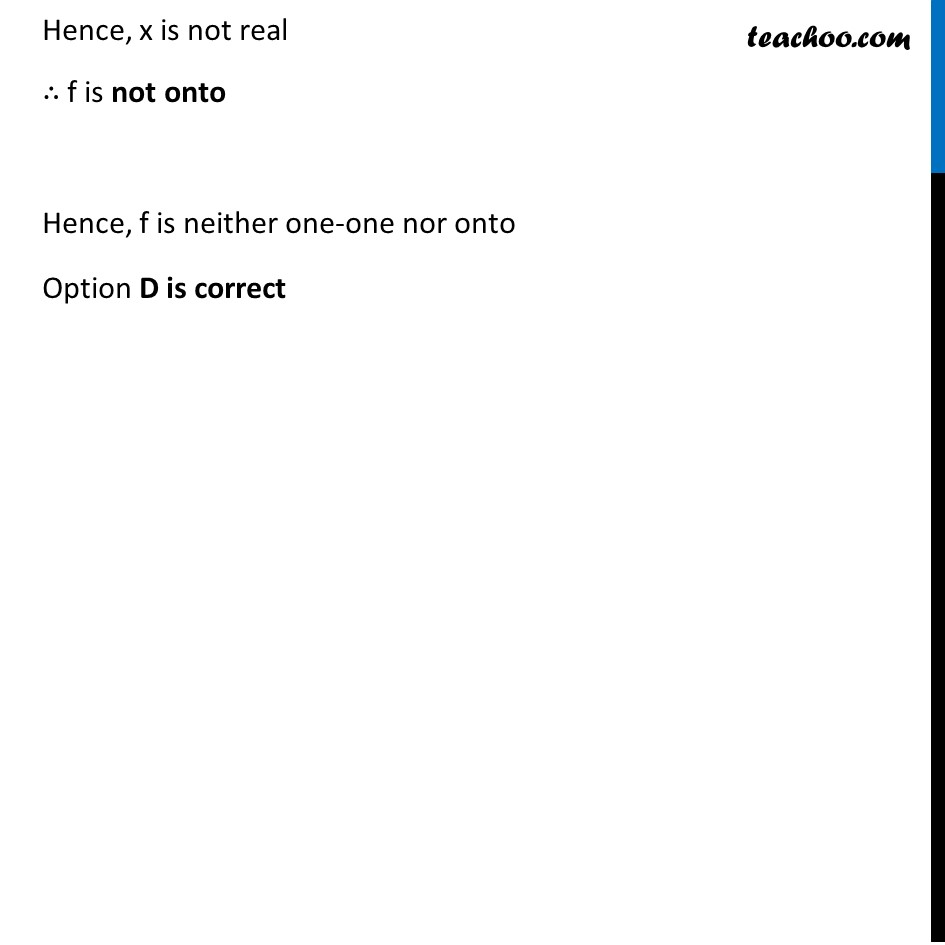Ex 1.2

Chapter 1 Class 12 Relation and Functions
Serial order wiseLearn in your speed, with individual attention - Teachoo Maths 1-on-1 Class

### Transcript

Ex 1.2, 11 Let f: R → R be defined as f(x) = x4. Choose the correct answer. (A) f is one-one onto (B) f is many-one onto (C) f is one-one but not onto (D) f is neither one-one nor onto f(x) = x4 Checking one-one f (x1) = (x1)4 f (x2) = (x2)4 Putting f (x1) = f (x2) (x1)4 = (x2)4 x1 = x2 or x1 = –x2Rough One-one Steps: 1. Calculate f(x1) 2. Calculate f(x2) 3. Putting f(x1) = f(x2) we have to prove x1 = x2 Since x1 does not have unique image, It is not one-one Example f(–1) = (–1)4 = 1 f(1) = (1)4 = 1 Here, f(–1) = f(1) , but –1 ≠ 1 Hence, it is not one-one Check onto f(x) = x4 Let f(x) = y , such that y ∈ R x4 = y x = ±𝑦^(1/4) Note that y is a real number, it can be negative also Putting y = −3 x = ±〖(−3)〗^(1/4) x = ± (√(−3))^(1/2) Which is not possible as root of negative number is not real Hence, x is not real ∴ f is not onto Hence, f is neither one-one nor onto Option D is correct